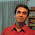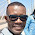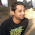## Friday, November 2, 2012

### Linked List implementation in Java

#### Here is a simple program which implements linked list in Java

Program
Class: Node.java
```package com.giri.linkedlist;

public class Node {
private int data;
private Node next;

public Node(int data) {
this.data = data;
}

public int getData() {
return data;
}

public void setData(int data) {
this.data = data;
}

public Node getNext() {
return next;
}

public void setNext(Node next) {
this.next = next;
}

public Node(int data, Node next) {
this.data = data;
this.next = next;
}

}
```
Program
Class: LinkedList.java
```package com.giri.linkedlist;

public class LinkedList {

private Node start;

public LinkedList() {
start = null;
}

public void insert(int x) {
if(start == null) {
start = new Node(x, start);
} else {
Node temp = start;

while(temp.getNext() != null) {
temp = temp.getNext();
}
Node newNode = new Node(x,null);
temp.setNext(newNode);
}
}

public void display() {
int count = 0;

if(start == null) {
System.out.println("\n List is empty !!");
} else {
Node temp = start;

while(temp.getNext() != null) {
System.out.println("count("+count+") , node value="+temp.getData());
count++;
temp = temp.getNext();
}
}
}

public void delete(int x) {
Node previous =  start;
Node temp = start;

while(temp.getData() != x) {
if(temp.getNext() == null) {
System.out.println("\nElement "+ x + " not found !");
break;
}

previous = temp;
temp = temp.getNext();

}
if(temp == start) {
start = start.getNext();
} else {
previous.setNext(temp.getNext());
}
}
}
```
Program
Class: MainClass.java
```package com.giri.linkedlist;

public class MainClass {

public static void main(String[] args) {
LinkedList ll = new LinkedList();

ll.insert(10);
ll.insert(2);
ll.insert(3);
ll.insert(4);
ll.insert(7);
ll.insert(10);
ll.insert(11);
ll.insert(12);
ll.insert(13);

System.out.println("\n--- Display ---\n");
ll.display();

System.out.println("\n--- Deleting 7 ---\n");
ll.delete(7);

System.out.println("\n--- After Deleting 7 ---\n");
ll.display();

System.out.println("\n--- Inserting 100 ---\n");
ll.insert(100);

System.out.println("\n--- After Inserting 100 ---\n");
ll.display();
}

}
```
Output
```--- Display ---

count(0) , node value=10
count(1) , node value=2
count(2) , node value=3
count(3) , node value=4
count(4) , node value=7
count(5) , node value=10
count(6) , node value=11
count(7) , node value=12

--- Deleting 7 ---

--- After Deleting 7 ---

count(0) , node value=10
count(1) , node value=2
count(2) , node value=3
count(3) , node value=4
count(4) , node value=10
count(5) , node value=11
count(6) , node value=12

--- Inserting 100 ---

--- After Inserting 100 ---

count(0) , node value=10
count(1) , node value=2
count(2) , node value=3
count(3) , node value=4
count(4) , node value=10
count(5) , node value=11
count(6) , node value=12
count(7) , node value=13
```

#### 9 comments :

1.Here in the Display function it should be changed as::

while(temp!=NULL) instead of while(temp.getNext!=NULL)

This will display all the elements in the list...otherwise your implementation leaves the last element from the list without displaying...Otherwise code works fine!

Thanks for posting it! Found it useful as a beginner :)

1.Thanks for that !

2.Thanks for that, i tried it by inserting 0 to 10 and it only printed up to 9.

2.there is an "error" in delete method..

3.What is the error in delete method?

4.there is an error in display as well ...in while it should be while(temp!=null)

5.LinkedList is based on indexes right?
Display and delete should be based on index
This is fine but to get exact implementation this code should be modified i guess
delete an obj will only delete one occurence
I am a newbie to this

6.delete function has bug. If element is not found and insert some new element later and display to see the stale output.

7.I propose the following changes to be done in the delete method. Please change variable type as per your needs.

public void deleteNode(Object key){

Node temp, previous;
temp=start;
previous=start;

if(start.getData()==key){
start=start.getNext();
}
else{

while(temp.getData() != key){
if(temp.getNext()!=null){
previous=temp;
temp=temp.getNext();
}
else{
return;
}
}
System.out.println("Data to be deleted" + temp.getData());
previous.setNext(temp.getNext());

}
}